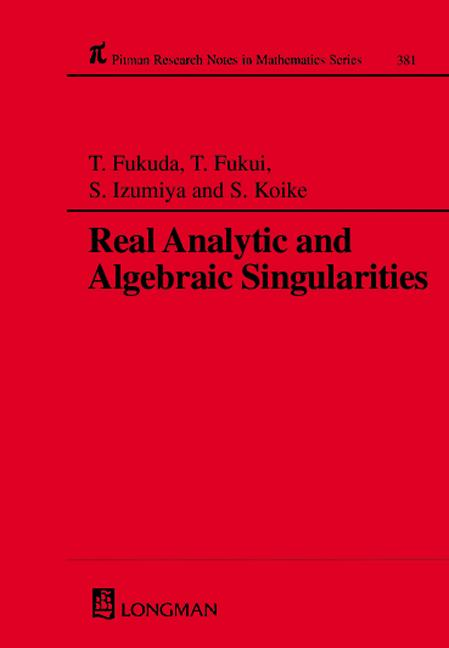# Real Analytic and Algebraic Singularities

## 1st Edition

Chapman and Hall/CRC

232 pages

##### Purchasing Options:\$ = USD
Hardback: 9780582328747
pub: 1997-12-12
Currently out of stock
\$185.00
x

FREE Standard Shipping!

### Description

This book contains a collection of papers covering recent progress in a number of areas of singularity theory. Topics include blow analyticity, recent progress in the research on equivalence relations of maps and functions, sufficiency of jets, and the transversality theorem. . Geometric and analytic studies of partial differential equations have been developed independently of one another, but the shock wave solutions appearing in natural phenomena are not well understood. Singularity theory may unify these studies and a survey based on this viewpoint is presented in which a new notion of weak solution is introduced. There are also reports on the recent progress in Zariski's conjecture on multiplicities of hypersurfaces, transcendency of analytic sets and on the topology of weighted homogeneous polynomials. This book will be of particular interest to specialists in singularities, partial differential equations, algebraic geometry and control theory.

Foreward

List of participants

Tzee-Char Kuo's contributions to real singularities

Blow-analytic equisingularities, properties, problems and progress

On blow-analytic equivalence of embedded curve singularities

On arc-analytic trivialization of singularities

Blow-analytic retraction onto the central fibre

An example of blow-analytic homeomorphism

The blow analytically constant stratum of real analytic singularities

Sufficiency of jets with respect to L-equivalence

A relative transversality theorem and its applications

Isomorphism of smooth map germs with isomorphic local algebra

Quelques proprietes de la distance geodesique

Relation between equivalence relations of maps and functions

Duality of the second fundamental form

Geometric study of quasilinear first order partial differnetial equations

Geometric approach to blow-up phenomena in non-linear problems

Multiplicity of complex analytic sets and bilipschitz maps

transcendence measures for subsets of local algebras

On topological invariance of weights for quasihomogeneous polynomials

Multiplicity as a C1 invariant

### Subject Categories

##### BISAC Subject Codes/Headings:
MAT007000
MATHEMATICS / Differential Equations
MAT022000
MATHEMATICS / Number Theory
MAT037000
MATHEMATICS / Functional Analysis